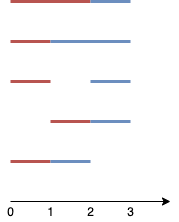# 大小为 K 的不重叠线段的数目

## 1621. 大小为 K 的不重叠线段的数目 (Medium)```输入：n = 4, k = 2

```输入：n = 3, k = 1

```

```输入：n = 30, k = 7

```

```输入：n = 5, k = 3

```

```输入：n = 3, k = 2

• `2 <= n <= 1000`
• `1 <= k <= n-1`

[动态规划]